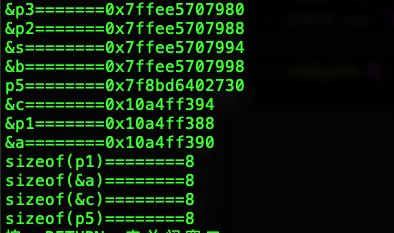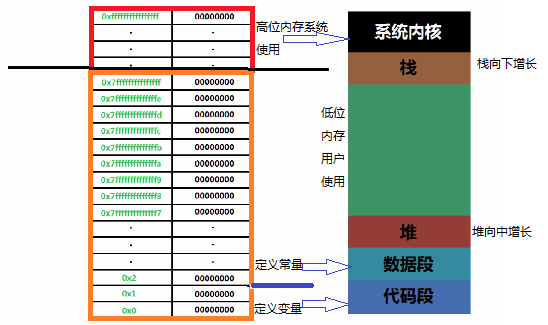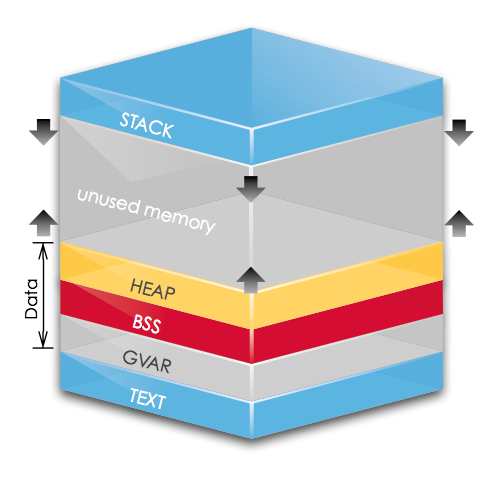## C++——程序的内存结构

https://blog.csdn.net/weixin_39540568/article/details/88363212

```#include <iostream>
#include <stdio.h>

using std::endl;
using std::cout;

char *p1; //全局未初始化区
int a = 0; //全局初始化区

int main()
{
int b; //栈
char s[] = "abc";//栈
char *p2;//栈
char *p3 = "123456";//  123456\0在常量区，p3在栈上。
static int c = 0; //全局（静态）初始化区
p1 = (char *)malloc(10);//堆
p2 = (char *)malloc(20);//椎
int *p5 = new int;//椎

cout << "&p3=======" << &p3 << endl;
cout << "&p2=======" << &p2 << endl;
cout << "&s========" << &s << endl;
cout << "&b========" << &b << endl;
cout << "p5========" << p5 << endl;
cout << "&c========" << &c << endl;
cout << "&p1=======" << &p1 << endl;
cout << "&a========" << &a << endl;

cout << "sizeof(p1)========" << sizeof(p1) << endl;
cout << "sizeof(&a)========" << sizeof(&a) << endl;
cout << "sizeof(&c)========" << sizeof(&c) << endl;
cout << "sizeof(p5)========" << sizeof(p5) << endl;

return 0;
}
```

MacOS 10.14.6的运行结果：## Text & Data & Bss（代码段、初始化数据、未初始化数据）

• .text： 也称为代码段(Code)，用来存放程序执行代码，同时也可能会包含一些常量(如一些字符串常量等）。该段内存为静态分配，只读(某些架构可能允许修改)。
这块内存是共享的,当有多个相同进程(Process)存在时，共用同一个text段。

• .data： 也有的地方叫GVAR(global value)，用来存放程序中已经初始化的非零全局变量。静态分配。

• data又可分为读写（RW）区域和只读（RO）区域。
-> RO段保存常量所以也被称为`.constdata` eg const数据
-> RW段则是普通非常全局变量，静态变量就在其中
• .bss： 存放程序中未初始化的和零值全局变量。静态分配，在程序开始时通常会被清零。

• 其中.bss和.data合称为数据段

text和data段都在可执行文件中，由系统从可执行文件中加载；而bss段不在可执行文件中，由系统初始化。

## 堆栈（Heap& Stack）

• Stack： 栈，存放Automatic Variables，按内存地址由高到低方向生长，其最大大小由编译时确定，速度快，但自由性差，最大空间不大。保存程序中的局部变量（也就是在代码块中的变量）这样的变量伴随着函数的调用和终止，在内存中也相应的增加或者减少。这样的变量在创建时期按顺序加入，在消亡的时候按相反的顺序移除。 （来自另一片博客解释：由编译器自动分配释放，存放函数的参数的值，局部变量的值等。在Windows下，栈是向低地址扩展的数据结构，是一块连续的内存的区 域。这句话的意思是栈顶的地址和栈的最大容量是系统预先规定好的，在WINDOWS下，栈的大小是2M(也有的是1M，总之是一个编译时就确定的常数)， 如果申请的空间超过栈的剩余空间时，将提示overflow。因此，能从栈获得的空间较小）

• Heap： 堆，自由申请的空间，按内存地址由低到高方向生长，其大小由系统内存/虚拟内存上限决定，速度较慢，但自由性大，可用空间大。 动态分配的内存在调用malloc（）或者相关函数产生，在调用free()时释放，由程序员而不是一系列固定的规则内存持续时间，因此内存块可在一个函数中创建，在另一个函数中释放。由于这点，动态内存分配所使用的部分可能就碎片，也就是说：在活动的内存块之间散布着未使用的内存片。动态分配内存往往要比栈分配的内存慢（一般由程序员分配释放，若程序员不释放，程序结束时可能由系统回收 。它与数据结构中的堆是两回事。堆是向高地址扩展的数据结构，是不连续的内存区域。这是由于系统是用链表来存储的空闲内存地址的，自然是不连续的，而链表 的遍历方向是由低地址向高地址。堆的大小受限于计算机系统中有效的虚拟内存。由此可见，堆获得的空间比较灵活，也比较大。）

• 每个线程都会有自己的栈，但是堆空间是共用的。

••

## 图解

sw-at 这张图中所示内存空间，地址由下往上增长，分别标示了 .text、.data、.bss、stack和heap的内存分部情况。